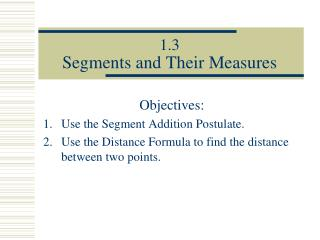# 1.3 Segments and Their Measures - PowerPoint PPT PresentationDownload Presentation1.3 Segments and Their Measures

1.3 Segments and Their MeasuresDownload Presentation## 1.3 Segments and Their Measures

- - - - - - - - - - - - - - - - - - - - - - - - - - - E N D - - - - - - - - - - - - - - - - - - - - - - - - - - -
##### Presentation Transcript

1. 1.3Segments and Their Measures Objectives: Use the Segment Addition Postulate. Use the Distance Formula to find the distance between two points.

2. Basic Vocabulary Postulate • a rule that is accepted as true without proof Theorem • a rule that must be proven true.

3. names of thepoints coordinates of thepoints The distance between points A and B or the length of AB Postulates • Ruler Postulate A B x1 x2 AB = | x2 – x1|

4. Postulates • Segment Addition Postulate If B is between A and C then AB + BC = AC A B C This indicates that the points are collinear.

5. AB BC AC Postulates • Segment Addition Postulate If B is between A and C then AB + BC = AC A B C The pieces of a segment add up to the whole segment.

6. AB BC AC Postulates • Segment Addition Postulate If A, B , and C are collinear and AB + BC = AC then B is between A and C A B C The postulate also works in reverse

7. Example 1: E D F

8. 9 35 Example 2: x E x D C B A

9. 2 20 Practice 1: x 2x D C B A

10. 425 yds 267 yds Example 3: Find the distance between Sue’s house and Jane’s house. 425 + 267 692 yds

11. 15 feet Example 4: A truck with a trailer has a total length of 27 feet. If the trailer has a length of 15 feet, how long is the truck? 12 feet 27 – 15 27 feet

12. y x Theorem The Distance Formula If A(x1, y1) and B(x2, y2) are points on a coordinate plane then the distance between A and B is A B C

13. the symbol for congruent this represents a number equal to a number this represents a figure the same size and shape as another figure Definition Congruent Segments segments that have the same length B Y A X

14. y x Example 5: Find the lengths of the segments. Are any of the segments congruent?

15. y x Example 5: Find the lengths of the segments. Are any of the segments congruent?

16. y x Example 5: none of the segments are congruent Find the lengths of the segments. Are any of the segments congruent?

17. Practice 3: Find the distance between the points. A (3, 1) B (0, 4)

18. Summary What is a postulate? Describe how to use the Segment Addition Postulate. Describe how to use the Distance Formula